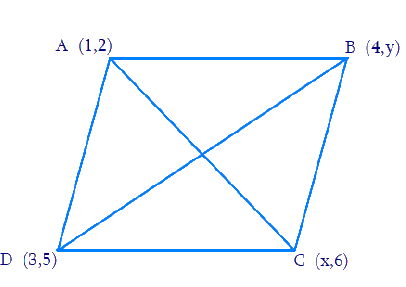# Ex.7.2 Q6 Coordinate Geometry Solution - NCERT Maths Class 10

Go back to  'Ex.7.2'

## Question

If $$(1, 2)$$, $$(4, y)$$, $$(x, 6)$$ and $$(3, 5)$$ are the vertices of a parallelogram taken in order, find $$x$$ and $$y$$.

## Text Solution

Reasoning:

The coordinates of the point $$P(x, y)$$ which divides the line segment joining the points $$A(x_1, y_1)$$ and $$B(x_2, y_2)$$, internally, in the ratio $$m_1 : m_2$$ is given by the Section Formula.

\begin{align}{{P(x,}}\,{{y)}} & \!=\! \left[\! {\frac{{{{m}}{{{x}}_2} \!+\! {{n}}{{{x}}_1}}}{{{{m}} \!+\! {{n}}}},\frac{{{{m}}{{{y}}_2} \!+\! {{n}}{{{y}}_1}}}{{{{m}} \!+\! {{n}}}}}\! \right]\end{align}

What is the known?

The $$x$$ and $$y$$ co-ordinates of the vertices of the parallelogram.

What is the unknown?

The missing $$x$$ and $$y$$ co-ordinate.

Steps:

From the Figure,Given,

• Let $$A (1, 2)$$, $$B (4, y)$$, $$C(x, 6)$$, and $$D(3, 5)$$ are the vertices of a parallelogram $$ABCD$$.
• Since the diagonals of a parallelogram bisect each other, Intersection point $$O$$ of diagonal $$AC$$ and $$BD$$ also divides these diagonals

Therefore, $$O$$ is the mid-point of $$AC$$ and $$BD$$.

If $$O$$ is the mid-point of $$AC$$, then the coordinates of $$O$$ are

\begin{align}\left( {\frac{{1 + x}}{2},\;\frac{{2 + 6}}{2}} \right) \Rightarrow \left( {\frac{{x + 1}}{2},\;4} \right)\end{align}

If $$O$$ is the mid-point of $$BD$$, then the coordinates of $$O$$ are

\begin{align}\left( {\frac{{4 + 3}}{2},\;\frac{{5 + y}}{2}} \right) \Rightarrow \left( {\frac{7}{2},\;\frac{{5 + y}}{2}} \right)\end{align}

Since both the coordinates are of the same point $$O$$,

\begin{align} &\therefore \frac{{x + 1}}{2} = \frac{7}{2}\;\;{\text{ and }}\;\;4 = \frac{{5 + y}}{2} \end{align}

\begin{align} &\Rightarrow x + 1 = 7\;\;{\text{ and }}\;\;5 + y = 8 \, \end{align} (By cross multiplying & transposing)

\begin{align} &\Rightarrow x = 6\;\;{\text{ and }}\;\; y = 3\end{align}

Learn from the best math teachers and top your exams

• Live one on one classroom and doubt clearing
• Practice worksheets in and after class for conceptual clarity
• Personalized curriculum to keep up with school